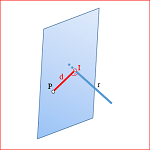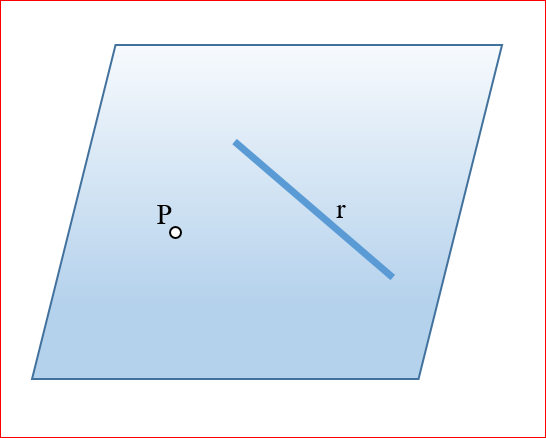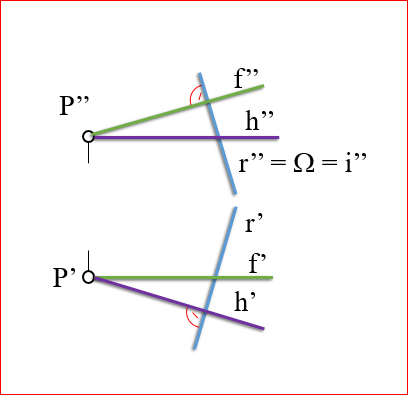# Diédrico System: Distance from a point to a lineWe can define distance from a point P to a straight r as the smallest of the distances from the point P the infinite points on the line r.

This problem can have two different approaches to determining the solution sought.

• Obtaining the plane perpendicular to the line, It is containing the point P
• Getting the plane containing the straight line and the point P

En el primer caso, to obtain the the plane perpendicular to the line containing the point P, we find the intersection (I) said plane with the line r. The distance will be the IP segment.

We can determine this distance obteniedo the line perpendicular to the line r from the point P and determine their point I intersection. Distance d of P to I It will be the minimum distance from this point to the line r.The second approach consists in determining a plane formed by the line and the point P.The solution will be determined by obtaining the line of the plane through P orthogonal to R.### Plane perpendicular to the line that contains the point P

A straight line is perpendicular to a plane if it is two nonparallel lines of said plane, thus, to determine the direction of a plane perpendicular to a straight need to determine two directions that are perpendicular to this straight. Then we will place the plane in space by passing through a given point and determine the intersection point (I) with the straight (r).We will use this approach in this article, leaving the reader to analyze the other approach we have discussed.

#### Plane perpendicular to a straight

We assume the proposed problem using two projections point and the line. In the figure the horizontal and vertical projections are shown in dihedral system of the line and the point.Determine the plane orthogonal to the line through the point obtaining a horizontal line and another front that can be passed directly through the point P, thereby we resolve the direction and position of the plane simultaneously searched.

The horizontal line, for being parallel to the horizontal plane H, It will be screened in this projection as a straight perpendicular a r. The segunada projection is perpendicular to the reference lines between the elevation (vertical projection) and plant (horizontal projection).

Similarly we get the straight front that, in the other case, será perpendicular a “r” in the vertical projection.Resolveremos la intersección utilizando un plano proyectante que contenga a la recta r, tal y como hemos visto en el capítuloIncidencias

En la figura se ha utilizado un plano Ω perpendicular al vertical de proyección que contenga a la recta “r”. Este plano seccionará al plano definido por la horizontal y la frontal según una recta “i” que coincidirá en la proyección vertical con el plano auxiliar Ω.Al determinar la proyección horizontal de la recta “i” podremos determinar el punto “I” buscado.La distancia en proyección será el segmento PIPara obtener la verdadera magnitud deberemos construir un triángulo rectángulo tal y como vimos al estudiar la “true magnitude of a line